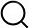# Also in the Article

Density functional calculations
This protocol is extracted from research article:
How heteroepitaxy occurs on strontium titanate

Procedure

The model surface structures discussed in this work were derived using density functional calculations based on the (linearized augmented plane wave) (L)APW+lo method as implemented in the WIEN2K code (55). Atomic positions were optimized using the modified Perdew-Burke-Ernzerhof functional (PBEsol) (56) implementation of the generalized gradient approximation. A 3D periodic surface slab model was created for each surface structure consisting of seven layers of the STO bulk (with a SrO layer surface termination) plus additional surface layers separated by 12 Å of vacuum, with inversion symmetry. Muffin-tin radii of 2.36, 1.90, 1.53, and 1.35 bohr were used for La, Sr, Ti, and O, respectively. The in-plane lattice parameter of these surface structures was based on the DFT-optimized bulk lattice parameter of STO (3.901 Å). All structures were relaxed until the force per atom, energy, and charge converged to within 0.1 eV/Å, 0.01 eV, and 0.005 e, respectively. The largest reciprocal lattice vector Kmax used in the plane wave expansion was given by $RMTminKmax=5.5$, where $RMTmin$ is the smallest muffin-tin radius. The k-meshes were given by a single k-point in the irreducible wedge of the first Brillouin zone.

Note: The content above has been extracted from a research article, so it may not display correctly.

Q&A